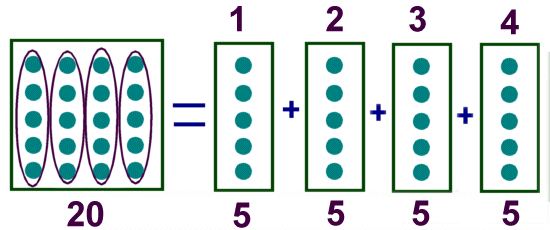# Kids Math

## Division Basics

What is division?

Division is breaking a number up into an equal number of parts.

Example:

20 divided by 4 = ?

If you take 20 things and put them into four equal sized groups, there will be 5 things in each group. The answer is 5.20 divided by 4 = 5.

Signs for Division

There are a number of signs that people may use to indicate division. The most common one is ÷, but the backslash / is also used. Sometimes people will write one number on top of another with a line between them. This is also called a fraction.

Example signs for "a divided by b":

a ÷ b
a/b
a
b

Dividend, Divisor, and Quotient

Each part of a division equation has a name. The three main names are the dividend, the divisor, and the quotient.
• Dividend - The dividend is the number you are dividing up
• Divisor - The divisor is the number you are dividing by
• Quotient - The quotient is the answer
Dividend ÷ Divisor = Quotient

Example:

In the problem 20 ÷ 4 = 5

Dividend = 20
Divisor = 4
Quotient = 5

Special Cases

There are three special cases to consider when dividing.

1) Dividing by 1: When dividing something by 1, the answer is the original number. In other words, if the divisor is 1 then the quotient equals the dividend.

Examples:

20 ÷ 1 = 20
14.7 ÷ 1 = 14.7

2) Dividing by 0: You cannot divide a number by 0. The answer to this question is undefined.

3) Dividend equals Divisor: If the dividend and the divisor are the same number (and not 0), then the answer is always 1.

Examples:

20 ÷ 20 = 1
14.7 ÷ 14.7 = 1

Remainder

If the answer to a division problem is not a whole number, the "leftovers" are called the remainder.

For example, if you were to try and divide 20 by 3 you would discover that 3 does not divide evenly into 20. The closest numbers to 20 that 3 can divide into are 18 and 21. You pick the closest number that 3 divides into that is smaller than 20. That is 18.

18 divided by 3 = 6, but there are still some leftovers. 20 -18 = 2. There are 2 remaining.

We write the remainder after an "r" in the answer.

20 ÷ 3 = 6 r 2

Examples:

12 ÷ 5 = 2 r 2
23 ÷ 4 = 5 r 3
18 ÷ 7 = 2 r 4

Division is the Opposite of Multiplication

Another way to think of division is as the opposite of multiplication. Taking the first example on this page:

20 ÷ 4 = 5

You can do the reverse, replacing the = with a x sign and the ÷ with an equal sign:

5 x 4 = 20

Examples:

12 ÷ 4 = 3
3 x 4 = 12

21 ÷ 3 = 7
7 x 3 = 21

Using multiplication is a great way to check your division work and get better scores on your math tests!

 Multiplication Intro to Multiplication Long Multiplication Multiplication Tips and Tricks Division Intro to Division Long Division Division Tips and Tricks Fractions Intro to Fractions Equivalent Fractions Simplifying and Reducing Fractions Adding and Subtracting Fractions Multiplying and Dividing Fractions Decimals Decimals Place Value Adding and Subtracting Decimals Multiplying and Dividing Decimals Statistics Mean, Median, Mode, and Range Picture Graphs Algebra Order of Operations Exponents Ratios Ratios, Fractions, and Percentages Geometry Polygons Quadrilaterals Triangles Pythagorean Theorem Circle Perimeter Surface Area Misc Basic Laws of Math Prime Numbers Roman Numerals Binary Numbers

Back to Kids Math

Back to Kids Study